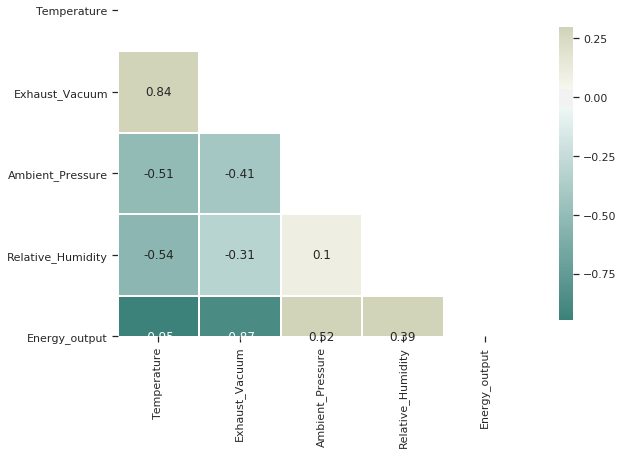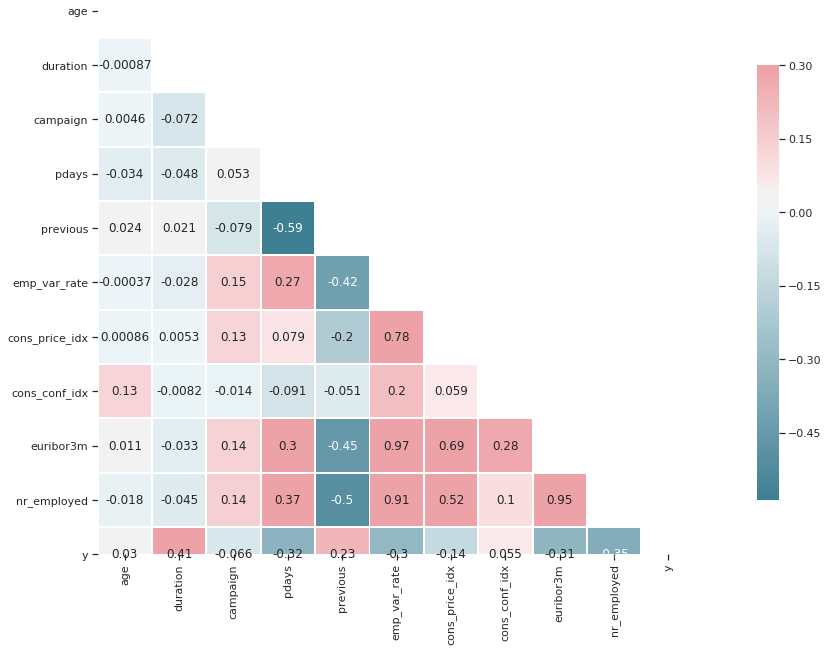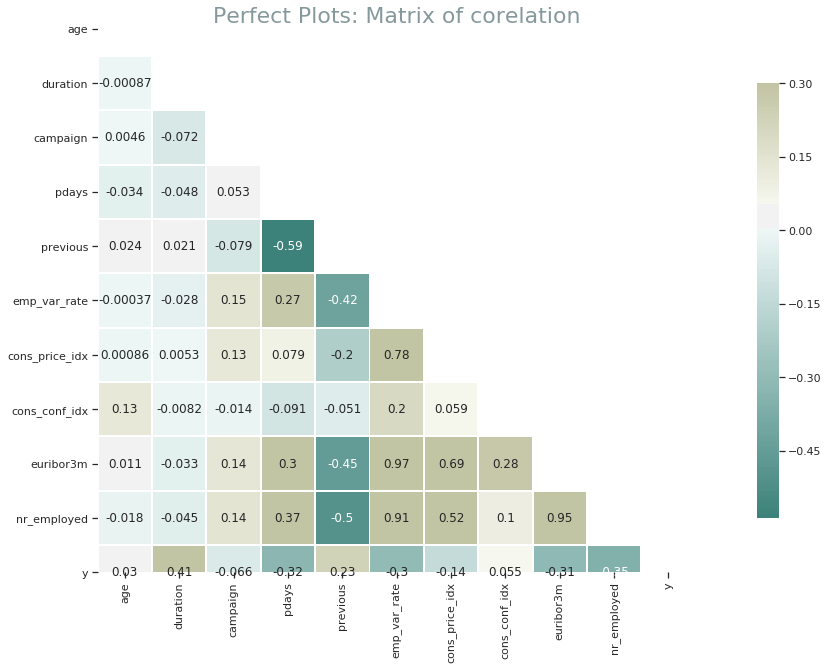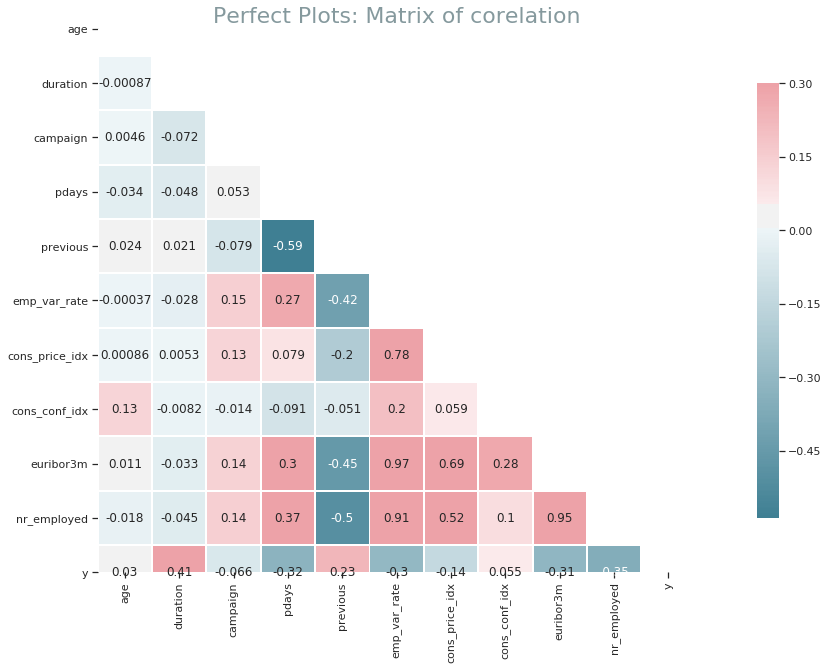# Perfect Plots_ Matrix of corelation

Feel free to read the code on GitHub

## Combined Cycle Power Plant Data Set¶

#### Data Set Information:¶

The dataset contains 9568 data points collected from a Combined Cycle Power Plant over 6 years (2006-2011), when the power plant was set to work with full load. Features consist of hourly average ambient variables Temperature (T), Ambient Pressure (AP), Relative Humidity (RH) and Exhaust Vacuum (V) to predict the net hourly electrical energy output (EP) of the plant.
A combined cycle power plant (CCPP) is composed of gas turbines (GT), steam turbines (ST) and heat recovery steam generators. In a CCPP, the electricity is generated by gas and steam turbines, which are combined in one cycle, and is transferred from one turbine to another. While the Vacuum is colected from and has effect on the Steam Turbine, he other three of the ambient variables effect the GT performance.
For comparability with our baseline studies, and to allow 5×2 fold statistical tests be carried out, we provide the data shuffled five times. For each shuffling 2-fold CV is carried out and the resulting 10 measurements are used for statistical testing.
We provide the data both in .ods and in .xlsx formats.

#### Attribute Information:¶

Features consist of hourly average ambient variables

• Temperature (T) in the range 1.81°C and 37.11°C,
• Ambient Pressure (AP) in the range 992.89-1033.30 milibar,
• Relative Humidity (RH) in the range 25.56% to 100.16%
• Exhaust Vacuum (V) in teh range 25.36-81.56 cm Hg
• Net hourly electrical energy output (EP) 420.26-495.76 MW
The averages are taken from various sensors located around the plant that record the ambient variables every second. The variables are given without normalization.
In :
import pandas as pd
import matplotlib.pyplot as plt
import seaborn as sns
import numpy as np

del df['Unnamed: 0']
df.columns = ['Temperature', 'Exhaust_Vacuum', 'Ambient_Pressure', 'Relative_Humidity', 'Energy_output']
df.sample(3)

Out:
Temperature Exhaust_Vacuum Ambient_Pressure Relative_Humidity Energy_output
7071 21.98 59.39 1015.25 84.52 446.79
1815 14.12 42.86 1011.84 88.29 471.86
5227 23.14 58.18 1008.89 81.82 444.51
In :
%matplotlib inline

sns.set(style="ticks")
corr = df.corr()

f, ax = plt.subplots(figsize=(12, 6))

cmap = sns.diverging_palette(180, 90, as_cmap=True)

square=True, linewidths=.9, cbar_kws={"shrink": .9})

Out:
<matplotlib.axes._subplots.AxesSubplot at 0x7fd267636d10>In :
df2 = pd.read_csv('/home/wojciech/Pulpit/1/bank.csv')
del df2['Unnamed: 0']
del df2['Unnamed: 0.1']

Out:
age job marital education default housing loan contact month day_of_week campaign pdays previous poutcome emp_var_rate cons_price_idx cons_conf_idx euribor3m nr_employed y
0 44 blue-collar married basic.4y unknown yes no cellular aug thu 1 999 0 nonexistent 1.4 93.444 -36.1 4.963 5228.1 0
1 53 technician married unknown no no no cellular nov fri 1 999 0 nonexistent -0.1 93.200 -42.0 4.021 5195.8 0
2 28 management single university.degree no yes no cellular jun thu 3 6 2 success -1.7 94.055 -39.8 0.729 4991.6 1
3 39 services married high.school no no no cellular apr fri 2 999 0 nonexistent -1.8 93.075 -47.1 1.405 5099.1 0
4 55 retired married basic.4y no yes no cellular aug fri 1 3 1 success -2.9 92.201 -31.4 0.869 5076.2 1

5 rows × 21 columns

In :
sns.set(style="ticks")

corr = df2.corr()

f, ax = plt.subplots(figsize=(22, 10))
cmap = sns.diverging_palette(580, 10, as_cmap=True)

square=True, linewidths=.9, cbar_kws={"shrink": 0.8})

Out:
<matplotlib.axes._subplots.AxesSubplot at 0x7fd2910ce590>### Definition¶

In :
def matrix_plot(df,title):

sns.set(style="ticks")

corr = df2.corr()

f, ax = plt.subplots(figsize=(22, 10))
#cmap = sns.diverging_palette(580, 10, as_cmap=True)
cmap = sns.diverging_palette(180, 90, as_cmap=True) #Inna paleta barw

square=True, linewidths=.9, cbar_kws={"shrink": 0.8})
plt.xticks(rotation=90)
plt.title(title,fontsize=22,color='#0c343d',alpha=0.5)
plt.show

In :
matrix_plot(df2, 'Perfect Plots: Matrix of corelation')## Definition by class¶

In :
class mx_plot:

def __init__(self,df,title):
self.df = df
self.title = title

def matrix(self):
sns.set(style="ticks")
corr = df2.corr()

f, ax = plt.subplots(figsize=(22, 10))
#cmap = sns.diverging_palette(580, 10, as_cmap=True)
cmap = sns.diverging_palette(580, 10, as_cmap=True) #Inna paleta barw

square=True, linewidths=.9, cbar_kws={"shrink": 0.8})
plt.xticks(rotation=90)
plt.title(title,fontsize=22,color='#0c343d',alpha=0.5)
plt.show

import seaborn as sns

In :
df=df2
title = 'Perfect Plots: Matrix of corelation'

PKP = mx_plot(df2,title)
PKP.matrix()